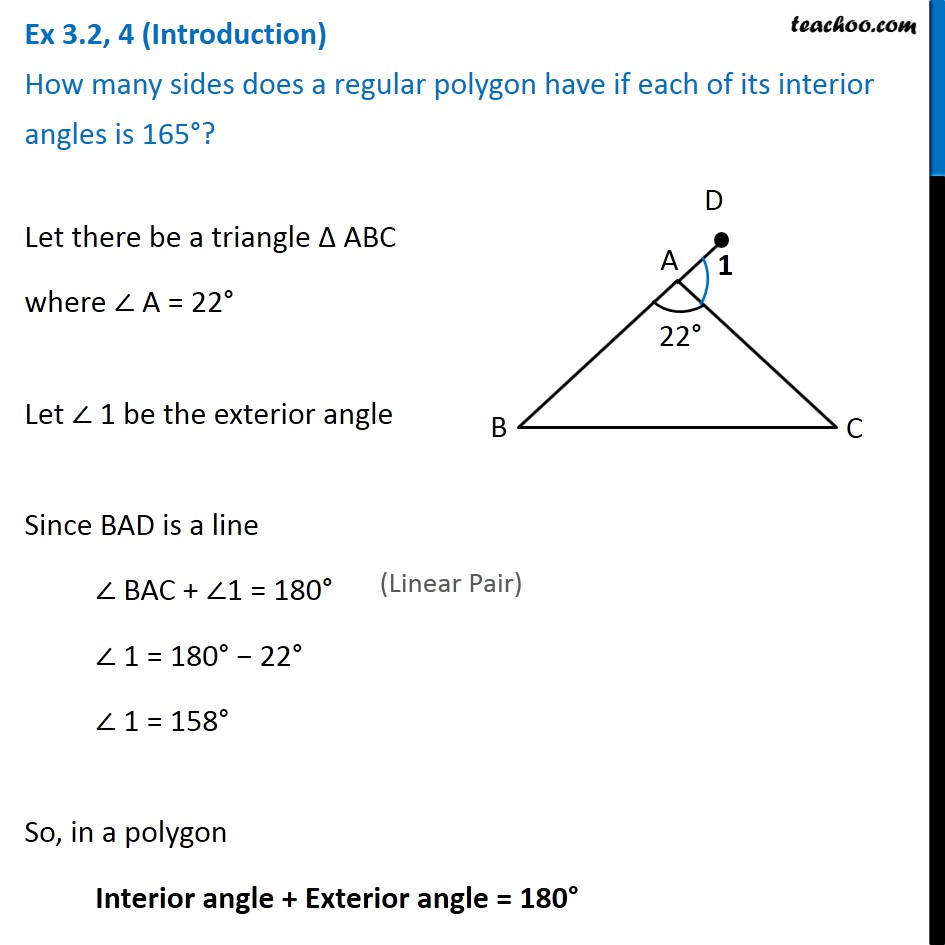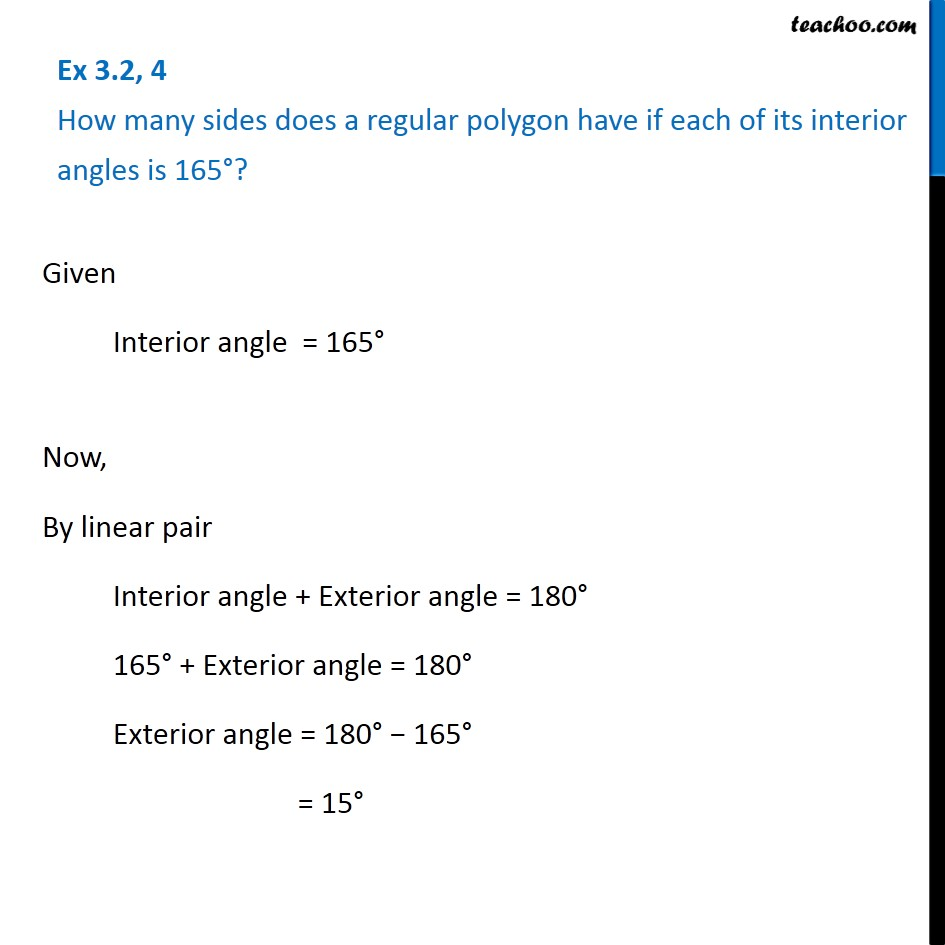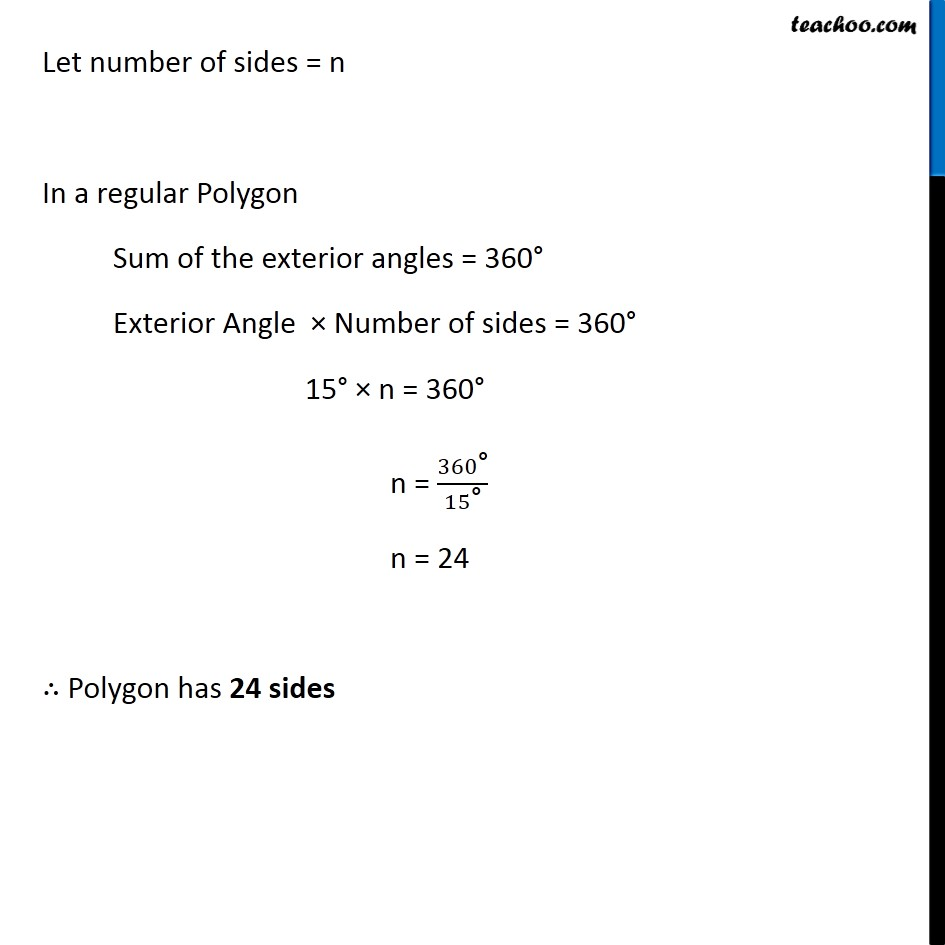Sum of Exterior Angles of PolygonsLearn in your speed, with individual attention - Teachoo Maths 1-on-1 Class

### Transcript

Ex 3.2, 4 (Introduction) How many sides does a regular polygon have if each of its interior angles is 165°?Let there be a triangle ∆ ABC where ∠ A = 22° Let ∠ 1 be the exterior angle Since BAD is a line ∠ BAC + ∠1 = 180° ∠ 1 = 180° − 22° ∠ 1 = 158° So, in a polygon Interior angle + Exterior angle = 180° Ex 3.2, 4 How many sides does a regular polygon have if each of its interior angles is 165°?Given Interior angle = 165° Now, By linear pair Interior angle + Exterior angle = 180° 165° + Exterior angle = 180° Exterior angle = 180° − 165° = 15° Let number of sides = n In a regular Polygon Sum of the exterior angles = 360° Exterior Angle × Number of sides = 360° 15° × n = 360° n = 360"°" /15"°" n = 24 ∴ Polygon has 24 sides